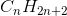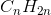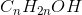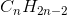Organic Chemistry : Help with Molecular Properties

Example Questions

← Previous 1 3 4

Example Question #241 : Organic Concepts

The degree of unsaturation for ephedrine (shown below) is __________.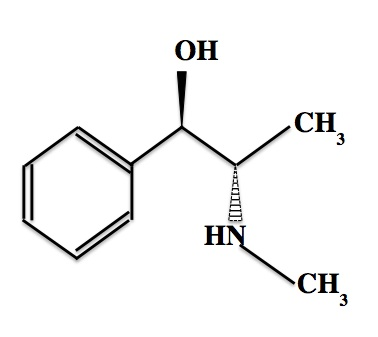Explanation:

The degree of unsaturation is equal to the number of rings plus the number of pi bonds in a molecule. Ephedrine has one ring and three pi bonds, so its degree of unsaturation is four.

To arrive at this answer, one could also use the formula below, whereis the number of carbon atoms,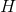is the number of hydrogen atoms,is the number of halogen atoms, andis the number of nitrogen atoms.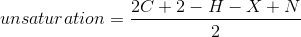For ephedrine,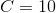,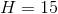,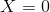, and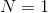.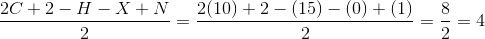Example Question #21 : Intermolecular Forces And Stability

Which of the following molecules are aromatic?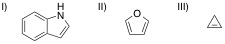III only

I and II

I, II, and III

II and III

I only

I and II

Explanation:

Aromaticity, a special type of energetic stabilization, occurs when the following criteria are met in a molecule:

1) The region in question is cyclic.

2) The region is planar, i.e., sp2 hybridization of all non-hydrogen atoms; molecule must be flat.

3) The total number of pi electrons in the system satisfies Hückel's rule, 4n +2.

Note: Hückel's rule confuses many students as they try to look for an "n" value to plug in. However, "n" simply represents any integer, such that n= 0, 1, 2, or 3 generate a total number of pi electrons of 2, 6, 10, or 14. To help, you could remember that benzene, the quintessential aromatic compound, has 6 pi electrons. Thus, you can add or subtract 4 from 6 to get a valid total number of pi electrons for an aromatic compound.

In the above question, I, indole, is an aromatic molecule as it is totally planar and features 10 total pi electrons. The trick in recognizing this molecule as an aromatic compound is remembering that the nitrogen atom, which would typically be assigned an sp3 hybridization, may rehybridize to sp2. This ensures a planar conformation and shifts its lone pair of electrons into a p orbital to contribute to the total pi electron count. This rehybridization is energetically favorable due to the energy gains associated with aromaticity.

II, furan, is aromatic by the same logic as I. The oxygen rehybridizes to offer up its p lone pair to a total count of 6 pi electrons.

III is not aromatic as it features a sp3 hybridized carbon.

Example Question #22 : Intermolecular Forces And Stability

Which of the given compounds has the highest boiling point?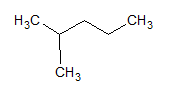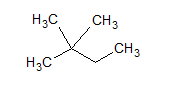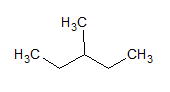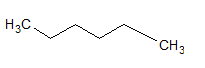Explanation:

Boiling point is affected by intermolecular forces, or the attractions between molecules. Molecules with higher intermolecular forces have higher boiling points. The type of intermolecular forces at play here depend largely on surface area of the molecule. Molecules with more surface area have greater attractive forces between them. The branched chain alkanes are more compact than the straight chain ones, so the straight chain alkane has the highest surface area. This means it has the greatest intermolecular forces, and thus the highest boiling point.

Note that increasing the length of the hydrocarbon chain and reducing branching will both raise the boiling point, while shortening the chain or increasing branching will lower the boiling point.

Example Question #23 : Intermolecular Forces And Stability

Which compound has the greatest dipole moment?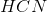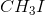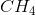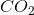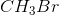Explanation:

The dipole moment refers to the difference in electronegativity between two atoms in a molecule. The greatest electronegativity difference results in the largest dipole moment. The trend for electronegativity increases as you move up and to the right on the periodic table. Moving to the right increases electronegativity far more than moving up.

The answer will be a molecule with a halogen, as the halogens are particularly electronegative. This narrows our options toor. Bromine is farther up the halogen group than iodine, so bromine will be more electronegative and result in the larger dipole moment. The correct answer is.

Example Question #24 : Intermolecular Forces And Stability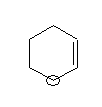What is the bond angle of the circled atom?

None of these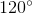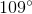Explanation:

The bond angle depends on the hybridization and structure of the atom. The circled atom has bonds to four single bonds (two carbons in the ring and two hydrogens). This means that it is sp3 hybridized. Because it has no lone pairs, the configuration of the atom and its bonds is a tetrahedral shape. The tetrahedral shape has bond angles of 109 degrees.

Example Question #25 : Intermolecular Forces And Stability

How many degrees of unsaturation does the given molecule have?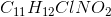Seven

Six

Five

None of these

Four

Six

Explanation:

An alkane with zero degrees of unsaturation has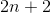hydrogens, whereis the number of carbons.

A halogen effectively is another hydrogen. An oxygen doesn't affect saturation. A nitrogen effectively subtracts a hydrogen. The molecule has one halogen, one nitrogen, two oxygens, and twelve hydrogens. This is effectively just 12 hydrogens.

A fully saturated 11-carbon alkane has 24 hydrogens. The given molecule effectively has 12 less hydrogens, which is equivalent to 6 degrees of unsaturation.

Example Question #26 : Intermolecular Forces And Stability

Which compound is the most stable?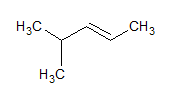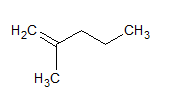All are very unstable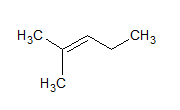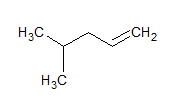Explanation:

Double bonds are most stable on carbons that are more highly substituted. The correct answer has the double bond on the tertiary and secondary carbon. The other choice that places the bond on a tertiary carbon also places it on the primary carbon. The name of the molecule that is most stable is 2-methyl-2-pentene.

Example Question #27 : Intermolecular Forces And Stability

How many degrees of unsaturation does the given compound have?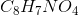Five

Two

Four

Six

Three

Six

Explanation:

A fully saturated hydrocarbon will havehydrogens, whereis the number of carbons in the compound.

A nitrogen is effectivelyhydrogen. Halogens are effectively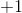. Oxygens are effectively. Seven hydrogens, a nitrogen, and four oxygens come out to a total of six.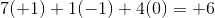This means that the difference between the actual saturation number and the fully saturated number is 12.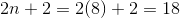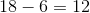To get degrees of saturation, you must divide this number by 2.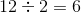There are six degrees of unsaturation.

Example Question #28 : Intermolecular Forces And Stability

Which of the following molecules is the most stable?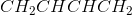Octane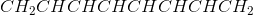ButeneExplanation:is the correct answer because it is the most conjugated molecule.is conjugated, but it is less conjugated thanand is therefore less stable. Neither octane nor butene are conjugated.

Example Question #29 : Intermolecular Forces And Stability

Which of the following is the correct general formula of an alkene?